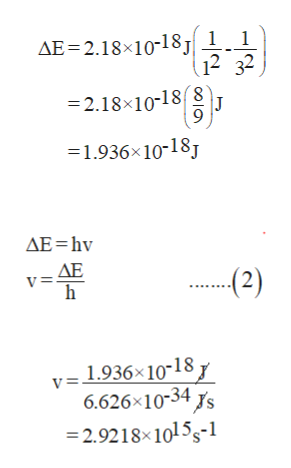# A hydrogen atom absorbs a photon of UV light and its electron enters the n = 3 energy level. Calculate the change in energy of the atom and the wavelength (in nm) of the photon.

Question
39 views
1. A hydrogen atom absorbs a photon of UV light and its electron enters the n = 3 energy level. Calculate the change in energy of the atom and the wavelength (in nm) of the photon.
check_circle

Step 1

Hydrogen atom absorbs UV radiation such that its electron gets excited from ground level and enters in 3rd energy levels.

The change in energy level can be calculated by using the given formula.

Step 2

Substitute 1 for n1 and 3 for n2 in equation (1).

Thus, the change in energy is 1.936 × 10-18 J.

Now for this, the frequency will be-

Substitut...help_outlineImage TranscriptioncloseAE 2.18x10-18J 1 1 32 =2.18x10-18 9 =1.936x10-18J AE hv AE v= (2) 1.936x10-18 6.626x10-34 s 2.9218 10155-1 v= fullscreen

### Want to see the full answer?

See Solution

#### Want to see this answer and more?

Solutions are written by subject experts who are available 24/7. Questions are typically answered within 1 hour.*

See Solution
*Response times may vary by subject and question.
Tagged in

### Atomic structure